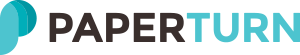# LGH User Guide 2018 UK 2nd Edition - Page 155Choke
Choke Reduction
Reduction
-- Page 50 --
Tension Calc Using Angles
-- Page 38 --
Tension
Calc
using angles
C hoker hitc h
R ated c apac ity
A ngle of c hoke R ated
c apac ity
in degrees
O ve r 120
100%
90 - 120
87%
60 - 89
74%
30 - 59
62%
0 - 29
49%
Horizontal
Angle
To determine the amount of tension
on a sling used at angles other than
90 degrees (vertical), use the table at
the right to obtain the Load Angle
Factor (L.A.F.) and following formula:
(Weight ÷ No of legs) x L.A.F.
Example: If the load weight is 4,000 kg, and
two (2) slings are used at a 40 degree angle
each.
(4000 ÷2) x 1.555 = 3,110
Therefore, each leg will have 3,110 kg of
tension.
RC = C x R
C : Choker rating
R : Rated Capacity
RC : Reduced choker rating
It is recommended that you
consider that only 2 legs will carry
the load, even when using 3 and 4
that all legs will carry an equal
Choker rating on tag: 5,000 kg
5000 x .49 = 2450
Reduced rating: 2,450 kg
Horizontal
Angle
Example
less than 30°
49% reduction
v5 - 5/16
ACRA Enterprises, Inc. 800-992-0689 www.acratech.com
Tension
Calc
Using Angles
Tension
Calc
using measurments
It's hard to determine the exact angle when working
following formula provides accurate calculations by
(Weight ÷ No of legs) X (S ÷ H)
Example: The load weight is 6,000 kg and two (2) slings are used.
You measure up the sling 36" (this is the "S" dimension) then
measure straight down and obtain a 24" measurment (this is the
"H" dimension).
(6000 ÷ 2) x (36 ÷ 24) = 4,500 kg of tension per leg
3000 x 1.5 = 4,500 kg of tension per leg
v5 - 5/16
-- Page 40 --
Drifting
To determine how much tension will be placed
upon chainfalls used in angular rigging
situations, use the following formula:
Tension on Chainfall "A" =
(Load weight × D2 × LA) ÷ (H × D3)
Tension on Chainfall "B" =
(Load weight × D1 × LB) ÷ (H × D3)
D3
D1
D2
s
Ten
ion
It is recommended that you
consider that only 2 legs will carry
the load, even when using 3 and 4
on
H
S
H
LA
"
ll "A
You can also use the sling
length and the vertical
height
ll
fa
n
ai
nfa
i
Cha
that all legs will carry an equal
TECHNICAL INFO
n
n
Te
sio
on
"
"B
Ch
LB
NOTE: This formula assumes that
both chainfalls are positioned at the
same elevation.
-- Page 39 --
v5 - 5/16
www.lgh.co.uk | 03704 247 247
155

Powered bySearch
Overview